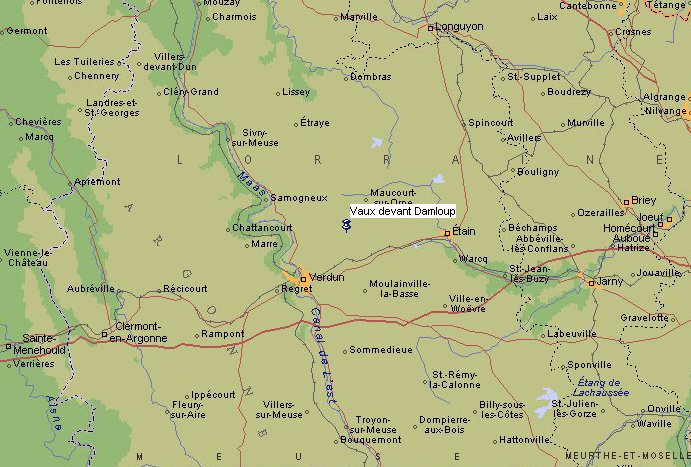# Francois Proth

## (1852 - 1879)

### "Vaux devant Damloup" near Verdun in France.### PROTH' S THEOREM (1878):

Let N = k*2n + 1 with k < 2n

If there is an integer a such that a((N-1)/2) = -1 (mod N), then N is prime !